# NetCDF 介绍

+关注继续查看
1. NetCDF
2. 1 NetCDF
3. 1.1概述（Overview）
4. NetCDF (network Common Data Form) is a set of software libraries and machine-independent data formats that support the creation, access, and sharing of array-oriented scientific data. Distributions are provided for Java and C/C++/Fortran. See the netCDF web site for more information.
5. NetCDF全称为network Common Data Format（ “网络通用数据格式”），是一个软件库与机器无关的数据格式，支持创建，访问基于数组的科研数据。分别提供了对Java和C / C++ / Fortran语言。
6. 对程序员来说，它和zip、jpeg、bmp文件格式类似，都是一种文件格式的标准。netcdf文件开始的目的是用于存储气象科学中的数据，现在已经成为许多数据采集软件的生成文件的格式。
7.    从数学上来说，netcdf存储的数据就是一个多自变量的单值函数。用公式来说就是f(x,y,z,...)=value, 函数的自变量x,y,z等在netcdf中叫做维(dimension)或坐标轴(axix),函数值value在netcdf中叫做变量(Variables)。而自变量和函数值在物理学上的一些性质，比如计量单位(量纲)、物理学名称在netcdf中就叫属性(Attributes)。
8. 1.2 数组理解
9. NetCDF是以数组为基础来对数据读写操作的，所以需要对数组理解明白，我们接触的二维数组比较容易理解，如果是三维、四维等多维数组的理解：
10. 基础：
11.     //静态初始化
12.     int a[][] = {{1,2},{2,3}};
13.     //动态初始化
14.     int c[][] = new int[];
15.     int d[][] = new int;
16.     /*********多维数组声明和初始化是从高维到低维 以下都是语法错误的：
17.      *int b[] = {{1,2},{2,3}};
18.      *int c[][] = new int[][];
19.      *int c[][] = new int[];
20.      *********多维数组可以看成以高维数组作为元素的数组*/
21. 如：int c[][] = new int[];
22.     c = new int;
23.     c = new int;
24. 在内存中如下存放：
25.
26. 2 参考网站
27. http://www.unidata.ucar.edu/
28. 2.1 Unidata
30. Unidata is a diverse community of over 160 institutions vested in the common goal of sharing data, and tools to access and visualize that data. For 20 years Unidata has been providing data, tools, and support to enhance earth-system education and research. In an era of increasing data complexity, accessibility, and multidisciplinary integration, Unidata provides a rich set of services and tools.
31. Unidatay是一个多样化的社区。对通用数据共享，处理工具，数据访问，可视化等处理，近20年来Unidata已提供数据，工具和支持，以加强地球系统教育和研究。在一个日益复杂的数据，交通方便，和多学科融合的时代，Unidata提供的服务和丰富的工具集。
32. 2.2.2 DATA
33. The Unidata Program helps researchers and educators acquire and use earth-related data. Most of the data are provided in "real time" or "near-real time" — that is, the data are sent to participants almost as soon as the observations are made. Unidata is a data facilitator, not a data archive center. We provide a mechanism whereby educators and researchers, by participating in our Internet Data Distribution (IDD) system, may subscribe to streams of current data that interest them.
34. Unidata项目帮助研究人员和教育工作者获得和使用地球相关的数据。大部分数据为实时数据或者临近数据，将数据发送到需要参与的对象。 Unidata是数据处理，而不是数据存储中心。
35. Available Data Types：（现有数据类型）
36. Forecast Model Output：出型预报
37. Satellite Data：卫星数据
39. Lightning Data：闪电数据
40. Wind Profiler Data：风分析的数据
41. Aircraft-Borne (ACARS)：航空传播数据
42. GPS Meteo. (SuomiNet)：GPS数据
43. …………
44. 3 NetCDF文件结构
45. 3.1 变量(Variables)
46. 变量对应着真实的物理数据。比如我们家里的电表，每个时刻显示的读数表示用户的到该时刻的耗电量。这个读数值就可以用netcdf里的变量来表示。它是一个以时间为自变量（或者说自变量个数为一维）的单值函数。再比如在气象学中要作出一个气压图，就是“东经xx度，北纬yy度的点的大气压值为多少帕”，这是一个二维单值函数，两维分别是经度和纬度。函数值为大气压。
47. 从上面的例子可以看出，netcdf中的变量就是一个N维数组，数组的维数就是实际问题中的自变量个数，数组的值就是观测得到的物理值。变量（数组值）在netcdf中的存储类型有六种，ascii字符(char) ,字节(byte), 短整型(short), 整型(int), 浮点(float), 双精度(double)。
48. 3.2 维(dimension)
49. 一个维对应着函数中的某个自变量，或者说函数图象中的一个坐标轴，在线性代数中就是一个N维向量的一个分量（这也是维这个名称的由来）。在netcdf中，一个维具有一个名字和范围（或者说长度，也就是数学上所说的定义域，可以是离散的点集合或者连续的区间）。在netcdf中,维的长度基本都是有限的，最多只能有一个具有无限长度的维。
50. 3.3 属性(Attribute)
51. 属性对变量值和维的具体物理含义的注释或者说解释。因为变量和维在netcdf中都只是无量纲的数字，要想让人们明白这些数字的具体含义，就得靠属性这个对象了。在netcdf中，属性由一个属性名和一个属性值（一般为字符串）组成。比如，在某个cdl文件(cdl文件的具体格式在下一节中讲述)中有这样的代码段：
52. temperature:units = "celsius" ;
53. 前面的temperature是一个已经定义好的变量（Variable），即温度，冒号后面的units就是属性名，表示物理单位，=后面的就是units这个属性的值，为“celsius” ，即摄氏度，整个一行代码的意思就是温度这个物理量的单位为celsius。
54. 3.4 表现形式（Representations）
55. Representations of netCDF CF(Climate and Forecast) Dimension and Variable Information:
56. CDL text, ncML, ncML with coordinate system extensions, and ncML-GML
57. 3.4.1 CDL
58. 网址：http://mailman.unidata.ucar.edu/software/netcdf/docs/netcdf/CDL-Syntax.html#CDL-Syntax
59.      netcdf example_1plus  {  // CDL with CF addions
60.      dimensions:         // dimension names and lengths are declared first
61.              lat = 5, lon = 10, level = 4, time = unlimited;
62.
63.      variables:          // variable types, names, shapes, attributes
64.              short   time(time);
65.                          time:standard_name  = "time";
66.                          time:units      = "hours since 1996-1-1";
67.              int     lat(lat), lon(lon), level(level);
68.                          lat:units       = "degrees_north";
69.                          lat:standard_name = "latitude";
70.                          lon:units       = "degrees_east";
71.                          lon:long_name = "longitude";
72.                          level:standard_name     = "air_pressure";
73.                          level:units     = "millibars";
74.              float   temp(time,level,lat,lon);
75.                          temp:long_name     = "temperature";
76.                          temp:standard_name     = "air_temperature";
77.                          temp:units         = "celsius";
78.              float   rh(time,lat,lon);
79.                          rh:long_name = "relative humidity";
80.                          rh:valid_range = 0.01.0;      // min and max
81.
82.              // global attributes
83.                          :source = "Fictional Model Output";
84.                          :Conventions = "CF-1.0";
85.
86.      data:                // optional data assignments
87.              level   = 1000850700500;
88.              lat     = 2030405060;
89.              lon     = -160,-140,-118,-96,-84,-52,-45,-35,-25,-15;
90.              time    = 12;
91.              rh      =.5,.2,.4,.2,.3,.2,.4,.5,.6,.7,
92.                       .1,.3,.1,.1,.1,.1,.5,.7,.8,.8,
93.                       .1,.2,.2,.2,.2,.5,.7,.8,.9,.9,
94.                       .1,.2,.3,.3,.3,.3,.7,.8,.9,.9,
95.                        0,.1,.2,.4,.4,.4,.4,.7,.9,.9;
96.      }
97. 如上：0.5表示time为12，lon为-160，lat为20的三维数据
98.       0.2表示time为12，lon为-140，lat为20的三维数据
99.       0  time为12，表示lon为-160，lat为60的三维数据
100. ………………….
101. 3.4.2 NCML
102. 参考网址：http://www.unidata.ucar.edu/software/netcdf/ncml/v2.2/Tutorial.html
103. <?xml version="1.0" encoding="UTF-8"?>
104. <netcdf xmlns="http://www.unidata.ucar.edu/namespaces/netcdf/ncml-2.2" location="T:/GALEON/SimpleSamples/SampleNetCDFbens.nc">
105.   <dimension name="lat" length="5" />
106.   <dimension name="lon" length="10" />
107.   <dimension name="level" length="4" />
108.   <dimension name="time" length="1" isUnlimited="true" />
109.   <attribute name="source" type="String" value="Fictional Model Output" />
110.   <attribute name="Conventions" type="String" value="CF-1.0" />
111.   <variable name="time" shape="time" type="short">
112.     <attribute name="standard_name" type="String" value="time" />
113.     <attribute name="units" type="String" value="hours since 1996-1-1" />
114.   </variable>
115.   <variable name="lat" shape="lat" type="int">
116.     <attribute name="units" type="String" value="degrees_north" />
117.     <attribute name="standard_name" type="String" value="latitude" />
118.   </variable>
119.   <variable name="lon" shape="lon" type="int">
120.     <attribute name="units" type="String" value="degrees_east" />
121.     <attribute name="long_name" type="String" value="longitude" />
122.   </variable>
123.   <variable name="level" shape="level" type="int">
124.     <attribute name="standard_name" type="String" value="air_pressure" />
125.     <attribute name="units" type="String" value="millibars" />
126.   </variable>
127.   <variable name="temp" shape="time level lat lon" type="float">
128.     <attribute name="long_name" type="String" value="temperature" />
129.     <attribute name="standard_name" type="String" value="air_temperature" />
130.     <attribute name="units" type="String" value="celsius" />
131.   </variable>
132.   <variable name="rh" shape="time lat lon" type="float">
133.     <attribute name="long_name" type="String" value="relative humidity" />
134.     <attribute name="valid_range" type="double" value="0.0 1.0" />
135.   </variable>
136. </netcdf>
137. 3.4.3 NCML-CS
138. 参考网址：http://www.unidata.ucar.edu/projects/THREDDS/GALEON/NetCDFandStandards.htm
139. <?xml version="1.0" encoding="UTF-8"?>
140. <netcdf xmlns="http://www.ucar.edu/schemas/netcdf" xmlns:xsi="http://www.w3.org/2001/XMLSchema-instance" xsi:schemaLocation="http://www.ucar.edu/schemas/netcdf http://www.unidata.ucar.edu/schemas/netcdf-cs.xsd" uri="T:/GALEON/SimpleSamples/SampleNetCDFbens.nc">
141.   <dimension name="lat" length="5" />
142.   <dimension name="lon" length="10" />
143.   <dimension name="level" length="4" />
144.   <dimension name="time" length="1" isUnlimited="true" />
145.   <attribute name="source" type="String" value="Fictional Model Output" />
146.   <attribute name="Conventions" type="String" value="CF-1.0" />
147.   <coordinateAxis name="time" shape="time" type="short" units="hours since 1996-1-1" axisType="Time">
148.     <attribute name="standard_name" type="String" value="time" />
149.     <attribute name="units" type="String" value="hours since 1996-1-1" />
150.     <attribute name="_CoordinateAxisType" type="String" value="Time" />
151.   </coordinateAxis>
152.   <coordinateAxis name="lat" shape="lat" type="int" units="degrees_north" axisType="Lat">
153.     <attribute name="units" type="String" value="degrees_north" />
154.     <attribute name="standard_name" type="String" value="latitude" />
155.     <attribute name="_CoordinateAxisType" type="String" value="Lat" />
156.   </coordinateAxis>
157.   <coordinateAxis name="lon" shape="lon" type="int" units="degrees_east" axisType="Lon">
158.     <attribute name="units" type="String" value="degrees_east" />
159.     <attribute name="long_name" type="String" value="longitude" />
160.     <attribute name="_CoordinateAxisType" type="String" value="Lon" />
161.   </coordinateAxis>
162.   <coordinateAxis name="level" shape="level" type="int" units="millibars" axisType="Pressure">
163.     <attribute name="standard_name" type="String" value="air_pressure" />
164.     <attribute name="units" type="String" value="millibars" />
165.     <attribute name="_CoordinateAxisType" type="String" value="Pressure" />
166.   </coordinateAxis>
167.   <variable name="temp" shape="time level lat lon" type="float" coordinateSystems="time-level-lat-lon">
168.     <attribute name="long_name" type="String" value="temperature" />
169.     <attribute name="standard_name" type="String" value="air_temperature" />
170.     <attribute name="units" type="String" value="celsius" />
171.   </variable>
172.   <variable name="rh" shape="time lat lon" type="float" coordinateSystems="time-lat-lon">
173.     <attribute name="long_name" type="String" value="relative humidity" />
174.     <attribute name="valid_range" type="double" value="0.0 1.0" />
175.   </variable>
176.   <coordinateSystem name="time-level-lat-lon">
177.     <coordinateAxisRef ref="time" />
178.     <coordinateAxisRef ref="level" />
179.     <coordinateAxisRef ref="lat" />
180.     <coordinateAxisRef ref="lon" />
181.   </coordinateSystem>
182.   <coordinateSystem name="time-lat-lon">
183.     <coordinateAxisRef ref="time" />
184.     <coordinateAxisRef ref="lat" />
185.     <coordinateAxisRef ref="lon" />
186.   </coordinateSystem>
187. </netcdf>
1. 附件文档：
2.
3. 4 NetCDF Java
4. 4.1 概述（Overview）
5. 参考网址：http://www.unidata.ucar.edu/software/netcdf-java/documentation.htm
6. The NetCDF-Java library implements a Common Data Model (CDM), a generalization of the NetCDF, OpenDAP and HDF5 data models. The library is a prototype for the NetCDF-4 project, which provides a C language API for the "data access layer" of the CDM, on top of the HDF5 file format. The NetCDF-Java library is a 100% Java framework for reading netCDF and other file formats into the CDM, as well as writing to the netCDF-3 file format. The NetCDF-Java library also implements NcML, which allows you to add metadata to CDM datasets, as well as to create virtual datasets through aggregation.
7.
8. NetCDF-Java库实现了CDM(通用数据模型)，CDM包括NetCDF,OpenDAP,HDF5数据模型，它是NetCDF-4项目的一个原型，NetCDF-4项目是紧跟HDF5文件格式后采用C语言作为CDM的的数据访问层API。在CDM中NetCDF-Java包是完全用Java架构来读取NetCDF和其他格式文件，和写netCDF-3格式文件一样。它还实现了NCML，允许你为CDM数据集添加元数据，和通过运算生成实际数据一样。
9. 4.2 CDM（通用数据模型）
10. 参考网址：http://www.unidata.ucar.edu/software/netcdf-java/CDM/index.html
11. Unidata’s Common Data Model (CDM) is an abstract data model for scientific datasets. It merges the netCDF, OPeNDAP, and HDF5 data models to create a common API for many types of scientific data. The NetCDF Java library is an implementation of the CDM which can read many file formats besides netCDF. We call these CDM files, a shorthand for files that can be read by the NetCDF Java library and accessed through the CDM data model.
12.
13. Unidata社区的CDM(通用数据模型)是一个科学数据的抽象数据模型，它包括了NetCDF, OPeNDAP, HDF5数据模型并为不同类型的科学数据创建了一个通用的API。NetCDF Java库实现了CDM，CDM除了能读取NetCDF格式外还有其他类型文件，我们把这些CDM文件作为那些能被NetCDF Java库读取和访问的的文件的简称。
14.
15. 4.3 NetCDF-Java/CDM  Architecture
16.
17. 4.4 CDM-FILES
18. General: NetCDF, OPeNDAP, HDF5, NetCDF4, HDF4, HDF-EOS
19. Gridded: GRIB-1, GRIB-2, GEMPAK
21. Point: BUFR, ASCII
22. Satellite: DMSP, GINI, McIDAS AREA
23. Misc: GTOPO, Lightning, etc
24. Others in development (partial):
25. AVHRR, GPCP, GACP, SRB, SSMI, HIRS (NCDC)
26. 4.5 Data Access Layer Object Model
27.
28. 4.6 NetCDF举例
29. 4.6.1 下载
30. 下载地址: http://www.unidata.ucar.edu/software/netcdf-java/documentation.htm
32. 目前最新版本为4.2.20
33. 最新版本需要JDK6
34. 4.6.2  生成NC文件
35. package my.demo;
36.
37. import java.io.IOException;
38. import java.util.ArrayList;
39.
40. import ucar.ma2.Array;
41. import ucar.ma2.DataType;
42. import ucar.nc2.Dimension;
43. import ucar.nc2.NetcdfFileWriteable;
44.
45. public class CreateNetcdf {
46.     @SuppressWarnings("unchecked")
47.     public static void main(String[] args) throws Exception {
48.         String filename = "testWrite.nc";
49.         NetcdfFileWriteable ncfile = NetcdfFileWriteable.createNew(filename,true); // add
50.         // dimensions
52.         Dimension lonDim = ncfile.addDimension("lon"3); // define
53.         // Variable
54.         ArrayList dims = new ArrayList();
59.         // 1D
60.         // attribute
61.         // of
62.         // length
63.         // 3
64.         Array data = Array.factory(int.classnew int[] { 3 }, new int[] { 1,2,3 });
66.         // add a string-valued variable: char svar(80)
68.         dims = new ArrayList();
71.         // string array: char names(3, 80)
73.         ArrayList dima = new ArrayList();
77.         // how about a scalar variable?
79.         // global
80.         // attributes
87.         // the
88.         // file
89.         try {
90.             ncfile.create();
91.         } catch (IOException e) {
92.             System.err.println("ERROR creating file " + ncfile.getLocation()+ "\n" + e);
93.         }
94.     }
95. }
96. 会生成一个testWrite.nc文件，该文件不能直接打开，可以通过下载的包中netcdfUI-4.2.jar打开：
97.
98. 4.6.3 读取NC文件
99. package my.demo;
100.
101. import java.io.IOException;
102. import java.util.List;
103.
104. import ucar.nc2.Dimension;
105. import ucar.nc2.NetcdfFile;
106. import ucar.nc2.Variable;
107.
109.     public static void main(String[] args) {
110.         String filename = "D:\\work\\netcdf\\testWrite.nc";
111.         NetcdfFile ncfile = null;
112.         try {
113.             ncfile = NetcdfFile.open(filename);
114.
116.             List<Dimension> list =  ncfile.getDimensions();
117.             for(Dimension d : list){
118.                 System.out.println("name="+d.getName()+" length="+d.getLength());
119.             }
121.             List<Variable> variables = ncfile.getVariables();
122.             System.out.println();
123.             for(Variable v : variables){
124.                 System.out.println("name="+v.getName()+" NameAndDimension="+v.getNameAndDimensions()+" ElementSize="+v.getElementSize());
125.             }
126.
127.         } catch (IOException ioe) {
128.         } finally {
129.             if (null != ncfile)
130.                 try {
131.                     ncfile.close();
132.                 } catch (IOException ioe) {
133.                 }
134.         }
135.     }
136. }
137. 运行打印如下：
138. name=lat length=3
139. name=lon length=3
140. name=svar_len length=80
141. name=names length=3
142.
143. name=temperature NameAndDimension=temperature(lat=3, lon=3) ElementSize=8
144. name=svar NameAndDimension=svar(svar_len=80) ElementSize=1
145. name=names NameAndDimension=names(names=3, svar_len=80) ElementSize=1
146. name=scalar NameAndDimension=scalar ElementSize=8
147. 4.6.4 读写文件
148. package my.demo;
149.
150. import java.io.IOException;
151.
152. import ucar.ma2.ArrayDouble;
153. import ucar.ma2.Index;
154. import ucar.ma2.InvalidRangeException;
155. import ucar.nc2.Dimension;
156. import ucar.nc2.NetcdfFileWriteable;
157.
158. public class WriteDataToNetcdf {
159.
160.     /**
161.      * @param args
162.      * @throws IOException
163.      */
164.     public static void main(String[] args) throws IOException {
165.         NetcdfFileWriteable ncfile = NetcdfFileWriteable.openExisting("D:\\work\\netcdf\\testWrite.nc"true);
166.         Dimension latDim = ncfile.getDimensions().get(0);
167.         Dimension lonDim = ncfile.getDimensions().get(1);
168.         ArrayDouble A = new ArrayDouble.D2(latDim.getLength(), lonDim.getLength());
169.         int i, j;
170.         Index ima = A.getIndex();
171.         for (i = 0; i < latDim.getLength(); i++) {
172.             for (j = 0; j < lonDim.getLength(); j++) {
173.                 A.setDouble(ima.set(i, j), (double) (2));
174.             }
175.         }
176.         int[] origin = new int;
177.         try {
178.             ncfile.write("temperature", origin, A);
179.             ncfile.close();
180.         } catch (IOException e) {
181.             System.err.println("ERROR writing file");
182.         } catch (InvalidRangeException e) {
183.             e.printStackTrace();
184.         }
185.     }
186. }
187. 该方法为Variable temperature进行赋值，可以将修改后的testWrite.nc在netcdfUI-4.2.jar中查看：
188.    double temperature(lat=3, lon=3);
189.      :units = "K";
190.      :scale = 123// int
191.  data:
192.   {
193.     {2.02.02.0},
194.     {2.02.02.0},
195.     {2.02.02.0}
196.   }
197. 4.6.5 读取二维数据
199.  Variable v = ncfile.findVariable(varName);
200. Variable v = ncfile.findVariable(varName);
201.  Array data = v.read("0:2:1, 0:19:1");
202. package my.demo;
203.
204. import java.io.IOException;
205.
206. import ucar.ma2.Array;
207. import ucar.nc2.NCdumpW;
208. import ucar.nc2.NetcdfFile;
209. import ucar.nc2.Variable;
210.
212.     public static void main(String[] args) {
213.         String filename = "D:\\work\\netcdf\\testWrite.nc";
214.         NetcdfFile ncfile = null;
215.         try {
216.             ncfile = NetcdfFile.open(filename);
217.             //find variable
218.             String variable = "temperature";
219.             Variable varBean = ncfile.findVariable(variable);
220.             //Reading data from a Variable
221.             if(null != varBean) {
223.                 Array data = varBean.read("0:2:1, 0:2:1");
224.                 Array data1 = varBean.read("0:2:2, 0:2:2");
225.                 System.out.println("读取所有：\n"+NCdumpW.printArray(all, variable, null));
226.                 System.out.println("x轴从0到2 跨度为1 y轴从0到2 跨度为1：\n"+NCdumpW.printArray(data, variable, null));
227.                 System.out.println("x轴从0到2 跨度为2 y轴从0到2 跨度为2：\n"+NCdumpW.printArray(data1, variable, null));
228.             }
229.
230.             if(null != varBean) {
231.                 int[] origin = new int[] { 0 , 0};
232.                 int[] size = new int[] { 3,3};
233.                 Array data2D = varBean.read(origin, size);
234.                 System.out.println("读取所有：\n"+NCdumpW.printArray(data2D, variable, null));
235.             }
236.
237.             if(null != varBean) {
238.                 int[] origin = new int[] { 1 , 1};
239.                 int[] size = new int[] { 2,1};
240.                 Array data2D = varBean.read(origin, size);
241.                 System.out.println("读取从第二行第二列开始为起点x数量为1，y数量为2：\n"+NCdumpW.printArray(data2D, variable, null));
242.             }
243.             System.out.println("由此可得结论：维上的起点都以数组0开始，且阵列顺序在坐标中是从右至左\n如：int[] size = new int[] { 2,1}，1代表x轴，2代表的是y轴....");
244.         } catch (Exception ioe) {
245.             ioe.printStackTrace();
246.         } finally {
247.             if (null != ncfile)
248.                 try {
249.                     ncfile.close();
250.                 } catch (IOException ioe) {
251.                 }
252.         }
253.     }
254. }
255.
257. 读取所有：
258. temperature =
259.   {
260.     {0.01.02.0},
261.     {1.02.03.0},
262.     {2.03.04.0}
263.   }
264.
265. x轴从02 跨度为1 y轴从02 跨度为1：
266. temperature =
267.   {
268.     {0.01.02.0},
269.     {1.02.03.0},
270.     {2.03.04.0}
271.   }
272.
273. x轴从02 跨度为2 y轴从02 跨度为2：
274. temperature =
275.   {
276.     {0.02.0},
277.     {2.04.0}
278.   }
279.
280. 读取所有：
281. temperature =
282.   {
283.     {0.01.02.0},
284.     {1.02.03.0},
285.     {2.03.04.0}
286.   }
287.
288. 读取从第二行第二列开始为起点x数量为1，y数量为2：
289. temperature =
290.   {
291.     {2.0},
292.     {3.0}
293.   }
294.
295. 由此可得结论：维上的起点都以数组0开始，且阵列顺序在坐标中是从右至左
296. 如：int[] size = new int[] { 2,1}，1代表x轴，2代表的是y轴....
297. 4.6.6 多维NetCDF(三维)
298. 4.6.6.1 创建
299. 创建三维NetCDF文件：
300. package my.demo;
301. import java.io.IOException;
302. import java.util.ArrayList;
303. import ucar.ma2.Array;
304. import ucar.ma2.DataType;
305. import ucar.nc2.Dimension;
306. import ucar.nc2.NetcdfFileWriteable;
307. public class Create3DNetCDF {
308.     @SuppressWarnings("unchecked")
309.     public static void main(String[] args) throws Exception {
310.         String filename = "test3D.nc";
311.         NetcdfFileWriteable ncfile = NetcdfFileWriteable.createNew(filename,true); // add
314.         Dimension lonDim = ncfile.addDimension("lon"3); // define
315.         ArrayList dims = new ArrayList();
321.         Array data = Array.factory(int.classnew int[] { 3 }, new int[] { 1,2,3 });
323.         try {
324.             ncfile.create();
325.         } catch (IOException e) {
326.             System.err.println("ERROR creating file " + ncfile.getLocation()+ "\n" + e);
327.         }
328.     }
329. }
330. 4.6.6.2 写数据
331. package my.demo;
332. import java.io.IOException;
333. import ucar.ma2.ArrayDouble;
334. import ucar.ma2.Index;
335. import ucar.ma2.InvalidRangeException;
336. import ucar.nc2.Dimension;
337. import ucar.nc2.NetcdfFileWriteable;
338. public class Write3DNetCDF {
339.     public static void main(String[] args) throws IOException {
340.         NetcdfFileWriteable ncfile = NetcdfFileWriteable.openExisting("D:\\work\\netcdf\\test3D.nc"true);
341.         Dimension timeDim = ncfile.getDimensions().get(0);
342.         Dimension latDim = ncfile.getDimensions().get(1);
343.         Dimension lonDim = ncfile.getDimensions().get(2);
344.         ArrayDouble A = new ArrayDouble.D3(timeDim.getLength(),latDim.getLength(), lonDim.getLength());
345.         int k,i, j;
346.         Index ima = A.getIndex();
347.         for(k = 0; k < timeDim.getLength(); k++){
348.             for (i = 0; i < latDim.getLength(); i++) {
349.                 for (j = 0; j < lonDim.getLength(); j++) {
350.                     A.setDouble(ima.set(k,i,j), (double) (k+i+j));
351.                 }
352.             }
353.         }
354.         int[] origin = new int;
355.         try {
356.             ncfile.write("temperature", origin, A);
357.             ncfile.close();
358.         } catch (IOException e) {
359.             System.err.println("ERROR writing file");
360.         } catch (InvalidRangeException e) {
361.             e.printStackTrace();
362.         }
363.     }
364. }
365.
366. 对应的CDL格式如下：
367.    double temperature(time=2, lat=3, lon=3);
368.      :units = "K";
369.      :scale = 123// int
370.  data:
371.   {
372.     {
373.       {0.01.02.0},
374.       {1.02.03.0},
375.       {2.03.04.0}
376.     },
377.     {
378.       {1.02.03.0},
379.       {2.03.04.0},
380.       {3.04.05.0}
381.     }
382.   }
383. 4.6.6.3 读数据
384. package my.demo;
385. import java.io.IOException;
386. import ucar.ma2.Array;
387. import ucar.nc2.NCdumpW;
388. import ucar.nc2.NetcdfFile;
389. import ucar.nc2.Variable;
391.     public static void main(String[] args) {
392.         String filename = "D:\\work\\netcdf\\test3D.nc";
393.         NetcdfFile ncfile = null;
394.         try {
395.             ncfile = NetcdfFile.open(filename);
396.             String variable = "temperature";
397.             Variable varBean = ncfile.findVariable(variable);
399.             if(null != varBean) {
401.                 System.out.println("读取所有：\n"+NCdumpW.printArray(all, variable, null));
402.             }
403.             if(null != varBean) {
404.                 int[] origin = new int[] { 0,1,1};
405.                 int[] size = new int[] { 2,2,2};
406.                 Array data2D = varBean.read(origin, size);
407.                 System.out.println("读取从第一维的0开始,第二维从1开始,第三维从1开始,数量分别为2,2,2：\n"+NCdumpW.printArray(data2D, variable, null));
408.             }
409.             // invoke reduce trans 3D to 2D
410.             if(null != varBean) {
411.                 int[] origin = new int[] { 0,1,1};
412.                 int[] size = new int[] { 1,2,2};
413.                 Array data2D = varBean.read(origin, size).reduce().reduce();
414.                 System.out.println("读取从第一维的0开始,第二维从1开始,第三维从1开始,数量分别为1,2,2并转为二维：\n"+NCdumpW.printArray(data2D, variable, null));
415.             }
416.         } catch (Exception ioe) {
417.             ioe.printStackTrace();
418.         } finally {
419.             if (null != ncfile)
420.                 try {
421.                     ncfile.close();
422.                 } catch (IOException ioe) {
423.                 }
424.         }
425.     }
426. }
427. 打印：
428. 读取所有：
429. temperature =
430.   {
431.     {
432.       {0.01.02.0},
433.       {1.02.03.0},
434.       {2.03.04.0}
435.     },
436.     {
437.       {1.02.03.0},
438.       {2.03.04.0},
439.       {3.04.05.0}
440.     }
441.   }
442. 读取从第一维的0开始,第二维从1开始,第三维从1开始,数量分别为2,2,2：
443. temperature =
444.   {
445.     {
446.       {2.03.0},
447.       {3.04.0}
448.     },
449.     {
450.       {3.04.0},
451.       {4.05.0}
452.     }
453.   }
454.
455. 读取从第一维的0开始,第二维从1开始,第三维从1开始,数量分别为1,2,2并转为二维：
456. temperature =
457.   {
458.     {2.03.0},
459.     {3.04.0}
460.   }
461. 4.7 NetCDF-NCML(Modifying existing files)
462. 通过NCML标记语言可以对NetCDF文件修改
463. 参考网址：http://www.unidata.ucar.edu/software/netcdf/ncml/v2.2/Tutorial.html
464. 4.8 NCML- Aggregation
465. 通过NCML合并存在的多个NetCDF文件
466. 参考网站：http://www.unidata.ucar.edu/software/netcdf/ncml/v2.2/Aggregation.html
467. 4.9 NetCDF-IOSP(I/O Service Provide)
468. 参考网址：http://www.unidata.ucar.edu/software/netcdf-java/tutorial/IOSPoverview.html
469. 4.9.1 Overview
470. A client uses the NetcdfFile, NetcdfDataset, or one of the Scientific Feature Type APIs to read data from a CDM file. These provide a rich and sometimes complicated API to the client. Behind the scenes, when any of these APIs actually read from a dataset, however, they use a very much simpler interface, the I/O Service Provider or IOSP for short. The Netcdf Java library has many implementations of this interface, one for each different file format that it knows how to read. This design pattern is called a Service Provider.
471.
472. IOSPs are managed by the NetcdfFile class. When a client requests a dataset (by calling NetcdfFile.open), the file is opened as a ucar.unidata.io.RandomAccessFile (an improved version of java.io.RandomAccessFile). Each registered IOSP is then asked "is this your file?" by calling isValidFile( ucar.unidata.io.RandomAccessFile). The first one that returns true claims it. When you implement isValidFile() in your IOSP, it must be very fast and accurate.
473. 4.9.2 IOServiceProvider
474. package ucar.nc2.iosp;
475. import ucar.ma2.Section;
476. import ucar.ma2.InvalidRangeException;
477. import ucar.ma2.StructureDataIterator;
478. import ucar.nc2.ParsedSectionSpec;
479. import ucar.nc2.Structure;
480. import java.io.IOException;
481. import java.nio.channels.WritableByteChannel;
482. /**
483.  * This is the service provider interface for the low-level I/O access classes (read only).
484.  * This is only used by service implementors.
485.  *
486.  * The NetcdfFile class manages all registered IOServiceProvider classes.
487.  * When NetcdfFile.open() is called:
488.  * <ol>
489.  * <li> the file is opened as a ucar.unidata.io.RandomAccessFile;</li>
490.  * <li> the file is handed to the isValidFile() method of each registered
491.  * IOServiceProvider class (until one returns true, which means it can read the file).</li>
492.  * <li> the open() method on the resulting IOServiceProvider class is handed the file.</li>
493.  *
494.  * @see ucar.nc2.NetcdfFile#registerIOProvider(Class) ;
495.  *
496.  * @author caron
497.  */
498. public interface IOServiceProvider {
499.
500.    /**
501.     * Check if this is a valid file for this IOServiceProvider.
502.     * You must make this method thread safe, ie dont keep any state.
503.     *
504.     * @param raf RandomAccessFile
505.     * @return true if valid.
506.     * @throws java.io.IOException if read error
507.     */
508.   public boolean isValidFile( ucar.unidata.io.RandomAccessFile raf) throws IOException;
509. }
510. 其他方法见官网介绍或API文档。
511. 4.9.3 AbstractIOServiceProvider
512. Your implementataion class should extend ucar.nc2.iosp.AbstractIOServiceProvider. This provides default implementation of some of the methods, so minimally, you only have to implement 4 methods:
513. public class MyIosp extends ucar.nc2.iosp.AbstractIOServiceProvider {
514.  1)  public boolean isValidFile(RandomAccessFile raf) throws IOException {}
515.  2)  public void open(RandomAccessFile raf, NetcdfFile ncfile, CancelTask cancelTask) throws IOException {}
516.  3)  public Array readData(Variable v2, Section wantSection) throws IOException, InvalidRangeException {}
517.  4)  public void close() throws IOException {}
518.
519.  5)  public String getFileTypeId() {}
520.  5)  public String getFileTypeVersion() {}
521.  5)  public String getFileTypeDescription();
522. }
523. 4.9.4 IOSP-Example
524. 通过IOSP对数据处理生成NetCDF文件已经读取NetCDF数据例子：
525. 参考网址：
526. http://www.unidata.ucar.edu/software/netcdf-java/tutorial/index.html
527.
528. 图中的例子为雷电数据，卫星数据，雷达数据相关

529. 本文转自94cool博客园博客，原文链接：http://www.cnblogs.com/94cool/p/3160262.html，如需转载请自行联系原作者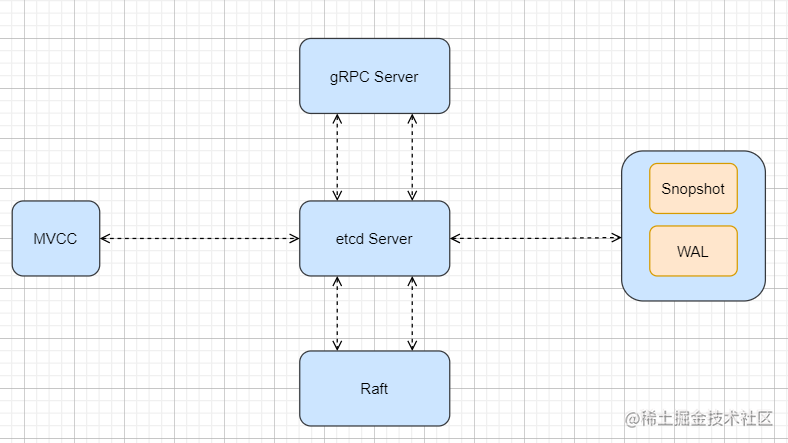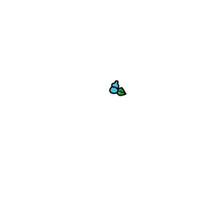Etcd 初步认识

76 0ETCD安全设置
ETCD安全设置
126 0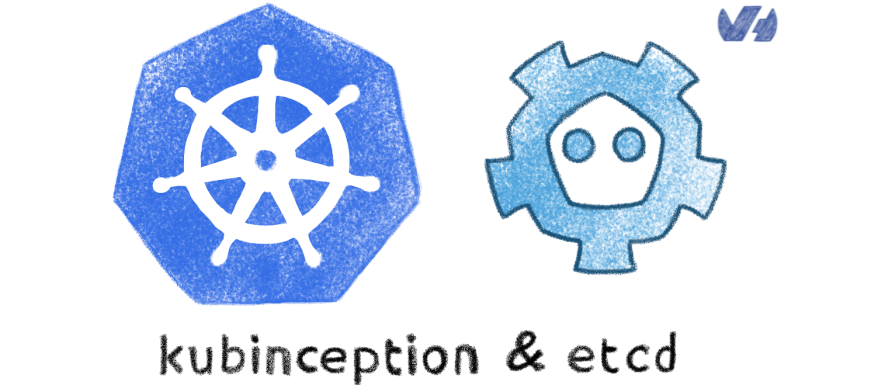836 0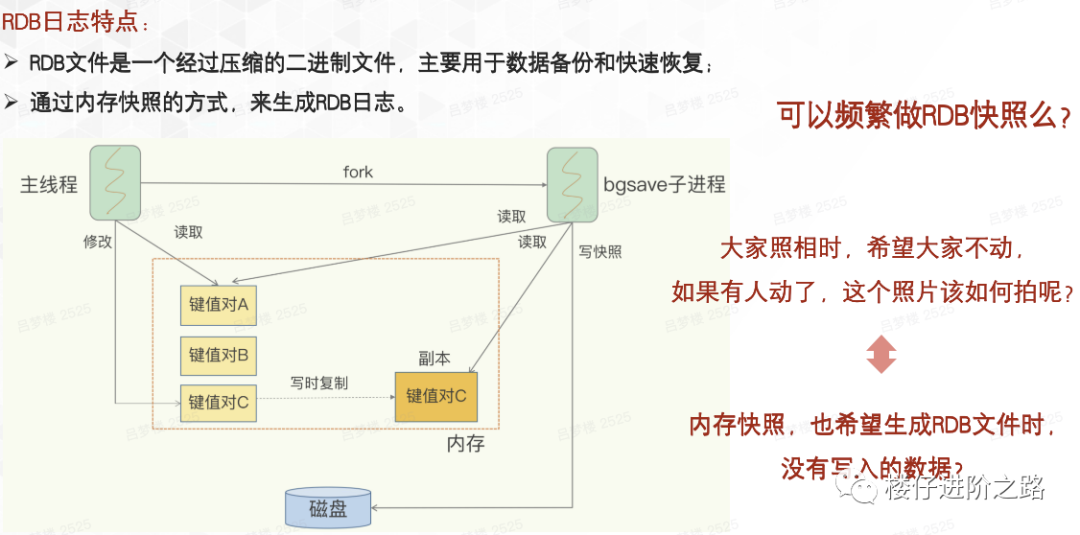ETCD教程-4.深入ETCD

439 0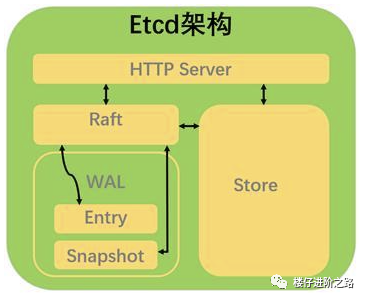ETCD教程-1.ETCD介绍
etcd是一个Go言编写的分布式、高可用的一致性键值存储系统，用于提供可靠的分布式键值存储、配置共享和服务发现等功能
393 0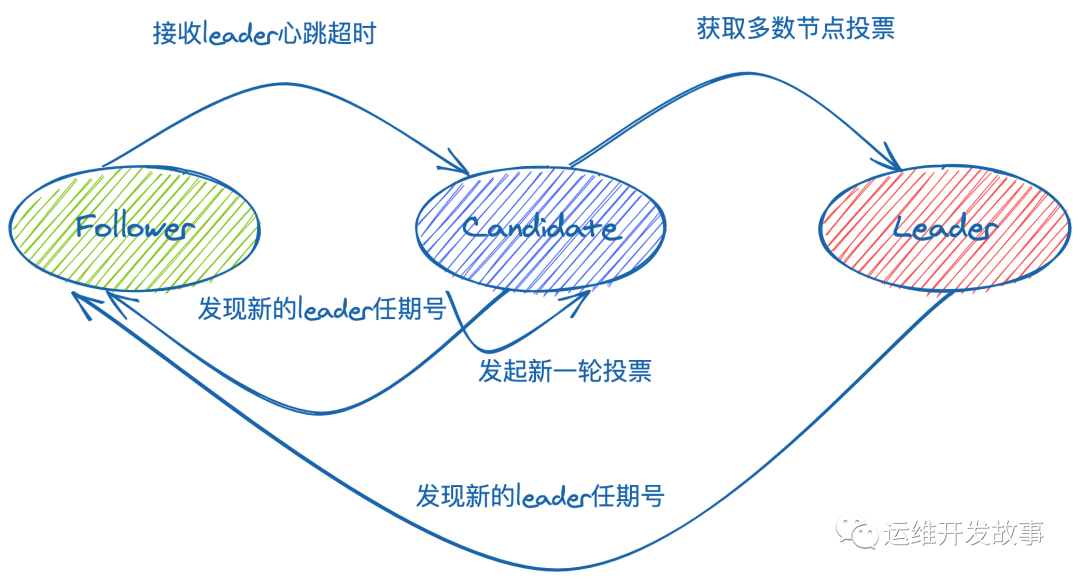etcd-raft学习
etcd-raft学习
56 0ETCD教程-3.ETCD体验

191 0Kubernetes：etcdctl3的常用命令
Kubernetes：etcdctl3的常用命令
302 0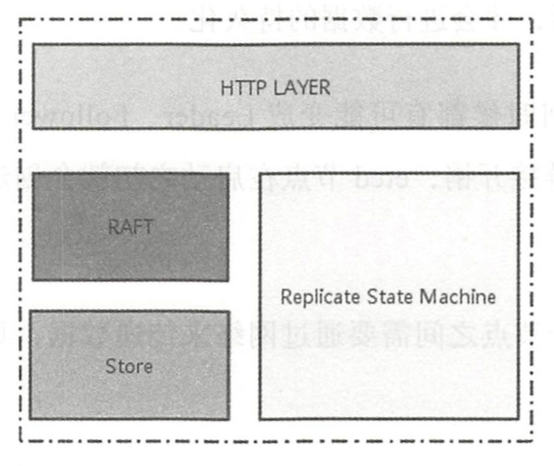etcd

428 0